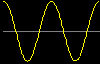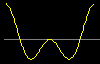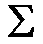# Harmonic Phasors and the Fourier Series

 Complicated periodic signals can be represented by a sum of cosine signals with frequencies that are integer multiples or "harmonics" of a fundamental frequency. This is the Fourier series.Note about viewing mpegs. Properties of a Fourier series can be demonstrated by viewing a cosine signal as the projection on the vertical axis of a rotating vector on the complex plane. Selecting this animation shows a cosine signal represented by a single phasor.A sum of cosine signals can be represented by projecting the vector sum of the individual phasors on the vertical axis. The vector sum is formed by placing the vectors in a "head-to-tail" fashion. Here is the sum of two cosines, the second wit h a nonzero phase angle.Here is a mathematical summary.